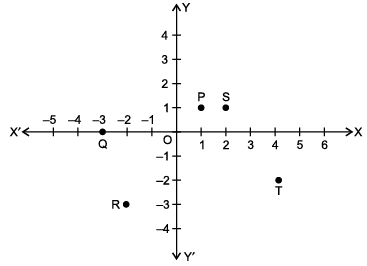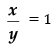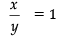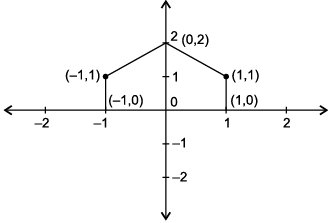# Test: Coordinate Geometry- Case Based Type Questions

## 10 Questions MCQ Test Mathematics (Maths) Class 9 | Test: Coordinate Geometry- Case Based Type Questions

Description
Attempt Test: Coordinate Geometry- Case Based Type Questions | 10 questions in 20 minutes | Mock test for Class 9 preparation | Free important questions MCQ to study Mathematics (Maths) Class 9 for Class 9 Exam | Download free PDF with solutions
QUESTION: 1

### Five friends playing a game in which they are standing at different positions, P, S, T, R andRohan is watching them playing. Few questions came to Rohan's mind while watching the game. Give answer to his questions by looking at the figure.Q. Name the point whose y-co-ordinate is zero :

Solution: As point Q lies on x-axis. Therefore, its y-coordinate is zero.
QUESTION: 2

### Five friends playing a game in which they are standing at different positions, P, S, T, R andRohan is watching them playing. Few questions came to Rohan's mind while watching the game. Give answer to his questions by looking at the figure.Q. Name the point lying in the third quadrant.

Solution: In third quadrant, both x-co-ordinate and y-coordinate are negative.
QUESTION: 3

### Five friends playing a game in which they are standing at different positions, P, S, T, R andRohan is watching them playing. Few questions came to Rohan's mind while watching the game. Give answer to his questions by looking at the figure.Q. What are the coordinates of P?

Solution: The Coordinate of P is (1, 1)
QUESTION: 4

Five friends playing a game in which they are standing at different positions, P, S, T, R andRohan is watching them playing. Few questions came to Rohan's mind while watching the game. Give answer to his questions by looking at the figure.

Q. Name the polygon formed on joining all these five points in an order.

Solution: Pentagon (5 sided polygon).
QUESTION: 5

Five friends playing a game in which they are standing at different positions, P, S, T, R andRohan is watching them playing. Few questions came to Rohan's mind while watching the game. Give answer to his questions by looking at the figure.

Q. (x, y) = (y, x), if :

Solution:Since,⇒ x = y

Also, (x, y) = (y, x), if x = y

QUESTION: 6

Sohan draws a gate of a temple on the graph paper. He has following points :

(– 1, 0), (1, 0), (1, 1), (– 1, 1) and (0, 2)Q. In which quadrant (– 1, 1) lies ?

Solution:

In 2nd quadrant, x-co-ordinate is negative and y-coordinate is positive.

QUESTION: 7

Sohan draws a gate of a temple on the graph paper. He has following points :

(– 1, 0), (1, 0), (1, 1), (– 1, 1) and (0, 2)Q. Write the abscissa of the point (0, 2).

Solution: x-co-ordinate of a point also called abscissa.
QUESTION: 8

Sohan draws a gate of a temple on the graph paper. He has following points :

(– 1, 0), (1, 0), (1, 1), (– 1, 1) and (0, 2)Q. Name the closed figure obtained.

Solution: A pentagon has five sides.
QUESTION: 9

Sohan draws a gate of a temple on the graph paper. He has following points :

(– 1, 0), (1, 0), (1, 1), (– 1, 1) and (0, 2)Q. Write the ordinate of the point (1, 0).

Solution: y-co-ordinate of a point also called ordinate.
QUESTION: 10

Sohan draws a gate of a temple on the graph paper. He has following points :

(– 1, 0), (1, 0), (1, 1), (– 1, 1) and (0, 2)Q. Which point from the following lies on Y-axis ?

Solution: On Y-axis, x-co-ordinate is zero.Use Code STAYHOME200 and get INR 200 additional OFF Use Coupon Code